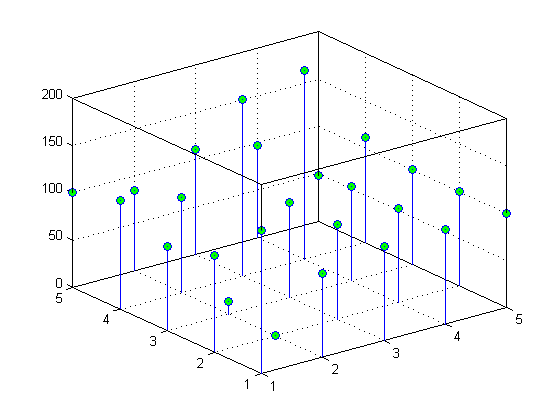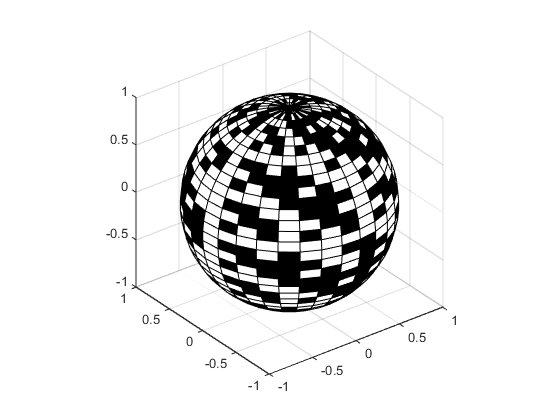## Representing Data as a Surface

### Functions for Plotting Data Grids

MATLAB® graphics defines a surface by the z-coordinates of points above a rectangular grid in the x-y plane. The plot is formed by joining adjacent points with straight lines. Surface plots are useful for visualizing matrices that are too large to display in numerical form and for graphing functions of two variables.

MATLAB can create different forms of surface plots. Mesh plots are wire-frame surfaces that color only the lines connecting the defining points. Surface plots display both the connecting lines and the faces of the surface in color. This table lists the various forms.

Function

Used to Create

`mesh`, `surf`

Surface plot

`meshc`, `surfc`

Surface plot with contour plot beneath it

`meshz`

Surface plot with curtain plot (reference plane)

`pcolor`

Flat surface plot (value is proportional only to color)

`surfl`

Surface plot illuminated from specified direction

`surface`

Low-level function (on which high-level functions are based) for creating surface graphics objects

### Functions for Gridding and Interpolating Data

These functions are useful when you need to restructure and interpolate data so that you can represent this data as a surface.

Function

Used to Create

`meshgrid`

Rectangular grid in 2-D and 3-D space

`griddata`

Interpolate scattered data

`griddedInterpolant`

Interpolant for gridded data

`scatteredInterpolant`

Interpolate scattered data

For a discussion of how to interpolate data, see Interpolating Gridded Data and Interpolating Scattered Data.

### Mesh and Surface Plots

The `mesh` and `surf` commands create 3-D surface plots of matrix data. If `Z` is a matrix for which the elements `Z(i,j)` define the height of a surface over an underlying `(i,j)` grid, then

```mesh(Z) ```

generates a colored, wire-frame view of the surface and displays it in a 3-D view. Similarly,

```surf(Z) ```

generates a colored, faceted view of the surface and displays it in a 3-D view. Ordinarily, the facets are quadrilaterals, each of which is a constant color, outlined with black mesh lines, but the `shading` command allows you to eliminate the mesh lines (`shading flat`) or to select interpolated shading across the facet (`shading interp`).

Surface object properties provide additional control over the visual appearance of the surface. You can specify edge line styles, vertex markers, face coloring, lighting characteristics, and so on.

### Visualizing Functions of Two Variables

1. To display a function of two variables, z = f(`x,y`), generate `X` and `Y` matrices consisting of repeated rows and columns, respectively, over the domain of the function. You will use these matrices to evaluate and graph the function.

2. The `meshgrid` function transforms the domain specified by two vectors, `x` and `y`, into matrices `X` and `Y`. You then use these matrices to evaluate functions of two variables: The rows of `X` are copies of the vector `x` and the columns of `Y` are copies of the vector `y`.

Example 1. Example: Illustrating the Use of meshgrid

To illustrate the use of `meshgrid`, consider the `sin(r)/r` or `sinc` function. To evaluate this function between -8 and 8 in both x and y, you need pass only one vector argument to `meshgrid`, which is then used in both directions.

```[X,Y] = meshgrid(-8:.5:8); R = sqrt(X.^2 + Y.^2) + eps; ```

The matrix `R` contains the distance from the center of the matrix, which is the origin. Adding `eps` prevents the divide by zero (in the next step) that produces `Inf` values in the data.

Forming the `sinc` function and plotting `Z` with `mesh` results in the 3-D surface.

```Z = sin(R)./R; figure mesh(X,Y,Z) ```#### Hidden Line Removal

By default, MATLAB removes lines that are hidden from view in mesh plots, even though the faces of the plot are not filled. You can disable hidden line removal and allow the faces of a mesh plot to be transparent with the `hidden` command:

`hidden off`#### Emphasizing Surface Shape

MATLAB provides a number of techniques that can enhance the information content of your graphs. For example, this graph of the `sinc` function uses the same data as the previous graph, but employs lighting, view adjustments, and a different colormap to emphasize the shape of the graphed function (`daspect`, `axis`, `view`, `camlight`).

```figure colormap hsv surf(X,Y,Z,'FaceColor','interp',... 'EdgeColor','none',... 'FaceLighting','gouraud') daspect([5 5 1]) axis tight view(-50,30) camlight left ```See the `surf` function for more information on surface plots.

### Surface Plots of Nonuniformly Sampled Data

You can use `meshgrid` to create a grid of uniformly sampled data points at which to evaluate and graph the `sinc` function. MATLAB then constructs the surface plot by connecting neighboring matrix elements to form a mesh of quadrilaterals.

To produce a surface plot from nonuniformly sampled data, use `scatteredInterpolant` to interpolate the values at uniformly spaced points, and then use `mesh` and `surf` in the usual way.

#### Example – Displaying Nonuniform Data on a Surface

This example evaluates the `sinc `function at random points within a specific range and then generates uniformly sampled data for display as a surface plot. The process involves these tasks:

• Use `linspace` to generate evenly spaced values over the range of your unevenly sampled data.

• Use `meshgrid` to generate the plotting grid with the output of `linspace`.

• Use `scatteredInterpolant` to interpolate the irregularly sampled data to the regularly spaced grid returned by `meshgrid`.

• Use a plotting function to display the data.

1. Generate unevenly sampled data within the range [-8, 8] and use it to evaluate the function:

```x = rand(100,1)*16 - 8; y = rand(100,1)*16 - 8; r = sqrt(x.^2 + y.^2) + eps; z = sin(r)./r; ```
2. The `linspace` function provides a convenient way to create uniformly spaced data with the desired number of elements. The following statements produce vectors over the range of the random data with the same resolution as that generated by the -8:.5:8 statement in the previous `sinc` example:

```xlin = linspace(min(x),max(x),33); ylin = linspace(min(y),max(y),33); ```
3. Now use these points to generate a uniformly spaced grid:

```[X,Y] = meshgrid(xlin,ylin); ```
4. The key to this process is to use `scatteredInterpolant` to interpolate the values of the function at the uniformly spaced points, based on the values of the function at the original data points (which are random in this example). This statement uses the default linear interpolation to generate the new data:

```f = scatteredInterpolant(x,y,z); Z = f(X,Y);```
5. Plot the interpolated and the nonuniform data to produce:

```figure mesh(X,Y,Z) %interpolated axis tight; hold on plot3(x,y,z,'.','MarkerSize',15) %nonuniform ```### Reshaping Data

Suppose you have a collection of data with the following (X, Y, Z) triplets:

XYZ
11152
2189
31100
41100
51100
12103
220
32100
42100
52100
1389
2313
33100
43100
53100
14115
24100
34187
44200
54111
15100
2585
35111
4597
5548

You can represent data that is in vector form using various MATLAB graph types, such as `surf`, `contour`, and `stem3`, by first restructuring the data. Use the (X, Y) values to define the coordinates in an x-y plane at which there is a Z value. The `reshape` and `transpose` functions can restructure your data so that the (X, Y, Z) triplets form a rectangular grid:

```x = reshape(X,5,5)'; y = reshape(Y,5,5)'; z = reshape(Z,5,5)';```

Reshaping results in three 5–by-5 arrays:

```x = 1 2 3 4 5 1 2 3 4 5 1 2 3 4 5 1 2 3 4 5 1 2 3 4 5 y = 1 1 1 1 1 2 2 2 2 2 3 3 3 3 3 4 4 4 4 4 5 5 5 5 5 z = 152 89 100 100 100 103 0 100 100 100 89 13 100 100 100 115 100 187 200 111 100 85 111 97 48```

You can now represent the values of Z with respect to X and Y. For example, create a 3–D stem graph:

`stem3(x,y,z,'MarkerFaceColor','g')`### Parametric Surfaces

The functions that draw surfaces can take two additional vector or matrix arguments to describe surfaces with specific x and y data. If `Z` is an m-by-n matrix, `x` is an n-vector, and `y` is an m-vector, then

```mesh(x,y,Z,C) ```

describes a mesh surface with vertices having color `C(i,j)` and located at the points

```(x(j), y(i), Z(i,j)) ```

where `x` corresponds to the columns of `Z` and `y` to its rows.

More generally, if `X`, `Y`, `Z`, and `C` are matrices of the same dimensions, then

```mesh(X,Y,Z,C) ```

describes a mesh surface with vertices having color `C(i,j)` and located at the points

```(X(i,j), Y(i,j), Z(i,j)) ```

This example uses spherical coordinates to draw a sphere and color it with the pattern of pluses and minuses in a Hadamard matrix, an orthogonal matrix used in signal processing coding theory. The vectors `theta` and `phi` are in the range `-`π ≤ `theta` ≤ π and `-`π`/2``phi` ≤ π`/2`. Because `theta` is a row vector and `phi` is a column vector, the multiplications that produce the matrices `X`, `Y`, and `Z` are vector outer products.

```figure k = 5; n = 2^k-1; theta = pi*(-n:2:n)/n; phi = (pi/2)*(-n:2:n)'/n; X = cos(phi)*cos(theta); Y = cos(phi)*sin(theta); Z = sin(phi)*ones(size(theta)); colormap([0 0 0;1 1 1]) C = hadamard(2^k); surf(X,Y,Z,C) axis square ```